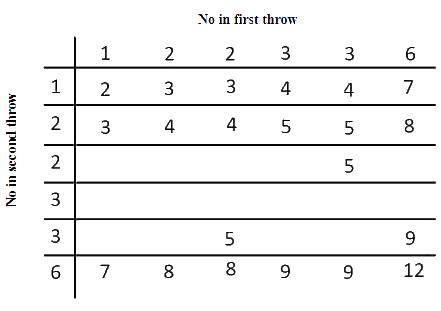# Ex.15.2 Q2 Probability Solution - NCERT Maths Class 10

Go back to  'Ex.15.2'

## Question

A die is numbered in such a way that its faces show the numbers $$1, 2, 2, 3, 3, 6$$. It is thrown two times and the total score in two throws is noted. Complete the following table which gives a few values of the total score on the two throws:What is the probability that the total score is (i) even? (ii) $$6$$? (iii) at least $$6$$?

Video Solution
Probability
Ex 15.2 | Question 2

## Text Solution

What is known?

A die is numbered in such a way that its faces show the numbers $$1, 2, 2, 3, 3, 6$$. It is thrown two times and the total score in two throws is noted

What is  unknown?

What is the probability that the total score is

(i) even?

(ii) $$6$$?

(iii) at least $$6$$?

Reasoning:

To solve this question, first find out the total number of outcomes and all the possible outcomes. Now, to find the probability use the formula given below

Probability

$=\frac{\begin{bmatrix} \text { Number of}\\ \text{ possible outcomes }\end{bmatrix} }{ \begin{bmatrix}\text { Total no of} \\ \text{favorable outcomes} \end{bmatrix} }$

Step:

 $$+$$ $$1$$ $$2$$ $$2$$ $$3$$ $$3$$ $$6$$ $$1$$ $$2$$ $$3$$ $$3$$ $$4$$ $$4$$ $$7$$ $$2$$ $$3$$ $$4$$ $$4$$ $$5$$ $$5$$ $$8$$ $$2$$ $$3$$ $$4$$ $$4$$ $$5$$ $$5$$ $$8$$ $$3$$ $$4$$ $$5$$ $$5$$ $$6$$ $$6$$ $$9$$ $$3$$ $$4$$ $$5$$ $$5$$ $$6$$ $$6$$ $$9$$ $$6$$ $$7$$ $$8$$ $$8$$ $$9$$ $$9$$ $$12$$

Total number of possible outcomes $$= 6 \times 6 =36$$

(i) No of possible outcomes when the sum is even $$= 18$$

Probability that the total score is even
\begin{align} & =\frac{\text{ No of possible outcomes }}{\text{ Total no of outcomes }} \\& =\frac{18}{36}\\&=\frac{1}{2} \\\end{align}

(ii) No of possible outcomes when the sum is $$6 = 4$$

Probability that of getting the sum $$6$$
\begin{align} & =\frac{\text{ No of possible outcomes }}{\text{ Total no of outcomes }} \\& =\frac{4}{36}\\&=\frac{1}{9}\end{align}

(iii) No of possible outcomes when the sum is at-least6(greater than $$5$$) $$= 15$$

Probability that of getting the sum at-least $$6$$
\begin{align} & =\frac{\text{No of possible outcomes }}{\text{Total no of outcomes}} \\& =\frac{15}{36}\\&=\frac{5}{12}\end{align}

Learn from the best math teachers and top your exams

• Live one on one classroom and doubt clearing
• Practice worksheets in and after class for conceptual clarity
• Personalized curriculum to keep up with school